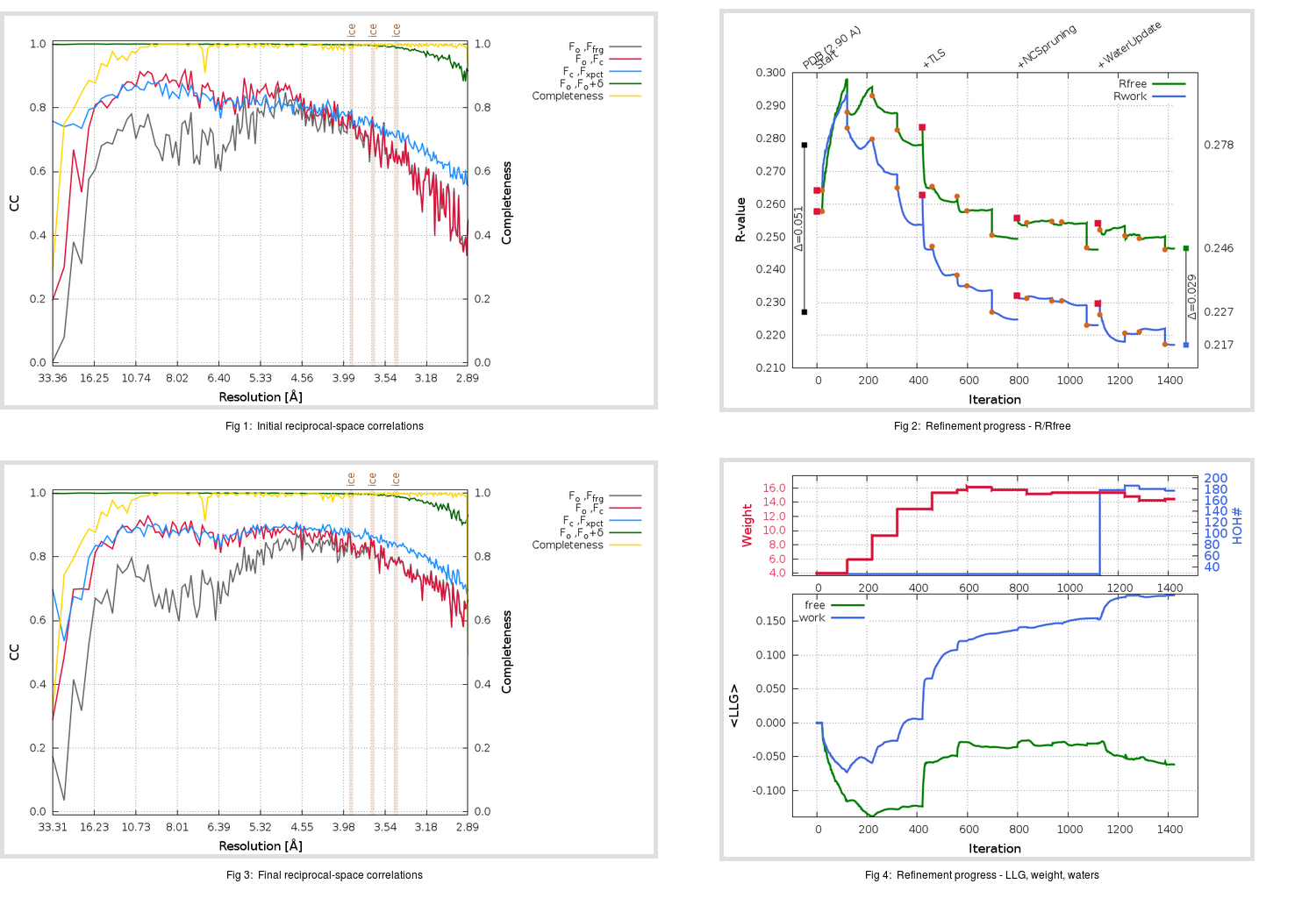Content:

```    Diffraction limits & principal axes of ellipsoid fitted to diffraction cut-off surface:
2.827         1.0000   0.0000   0.0000       0.992 a* - 0.130 c*
2.840         0.0000   1.0000   0.0000       b*
2.846         0.0000   0.0000   1.0000       c*
```

## Deposited

` `
 Date deposited Date data collection Resolution R, Rfree 20080501 2.90 0.2240 0.2780

Molprobity (CCP4 7.0 version) summary:

```Ramachandran outliers =   3.73 %
favored =  87.11 %
Rotamer outliers      =   8.64 %
C-beta deviations     =     0
Clashscore            =  18.87
RMS(bonds)            =   0.0105
RMS(angles)           =   1.26
MolProbity score      =   3.10
Resolution            =   2.90
R-work                =   0.2240
R-free                =   0.2780
```

```Number of waters      =    27

<B> (all atoms) =   84.36 ( sd =   23.45 ) for      12639 non-hydrogen atoms
<B>   (protein) =   84.19 ( sd =   23.29 ) for      12518 non-hydrogen atoms
<B>     (water) =   53.11 ( sd =    9.31 ) for         27 non-hydrogen atoms
<B>    (others) =  115.74 ( sd =   20.17 ) for         94 non-hydrogen atoms

B min/max       (all non-hydrogen atoms) =   28.93 /  157.27
B min/max   (protein non-hydrogen atoms) =   34.30 /  157.27
B min/max     (water non-hydrogen atoms) =   28.93 /   76.34
B min/max     (other non-hydrogen atoms) =   72.75 /  142.15
```

## BUSTER (re-)refinement

` `

Molprobity (CCP4 7.0 version) summary:

```Ramachandran outliers =   0.92 %
favored =  94.37 %
Rotamer outliers      =  10.41 %
C-beta deviations     =     9
Clashscore            =   6.29
RMS(bonds)            =   0.0118
RMS(angles)           =   1.61
MolProbity score      =   2.50
Resolution            =   2.89
R-work                =   0.2172
R-free                =   0.2465
```

```Number of waters      =   177

<B> (all atoms) =  101.86 ( sd =   30.30 ) for      12789 non-hydrogen atoms
<B>   (protein) =  101.78 ( sd =   29.85 ) for      12518 non-hydrogen atoms
<B>     (water) =   75.84 ( sd =   14.18 ) for        177 non-hydrogen atoms
<B>    (others) =  161.45 ( sd =   32.53 ) for         94 non-hydrogen atoms

B min/max       (all non-hydrogen atoms) =   47.67 /  210.91
B min/max   (protein non-hydrogen atoms) =   51.57 /  210.91
B min/max     (water non-hydrogen atoms) =   47.67 /  145.70
B min/max     (other non-hydrogen atoms) =   80.01 /  205.64
```

Refinement progression:Results:

` `
 File Remark 3D0I_aB_refine.01_04_refine.pdb.gz exact refinement commands are in header 3D0I_aB_refine.01_04_refine.mtz.gz including original deposited data and several re-refinement map coefficients 3D0I_aB_refine.01_04_BUSTER_model.cif.gz including any non-standard compound restraints 3D0I_aB_refine.01_04_BUSTER_refln.cif.gz